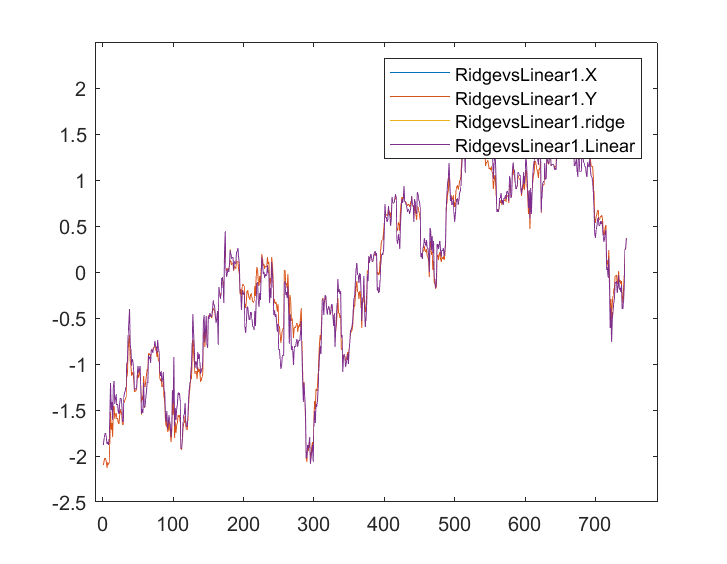# Discussion of article "Data Science and Machine Learning (Part 10): Ridge Regression"

New article Data Science and Machine Learning (Part 10): Ridge Regression has been published:

Ridge regression is a simple technique to reduce model complexity and prevent over-fitting which may result from simple linear regression.

The models have a slightly different performance when you use all the data as the training data.

When the outputs were stored and plotted in the same axis this is their graph;I can hardly see any difference between the Linear model to the predictor marked in blue, I can only see the difference between the two models and the ridge regression doesn't fit well to the dataset, that's good news. Let's train and test both of the models one by one.

Author: Omega J Msigwa